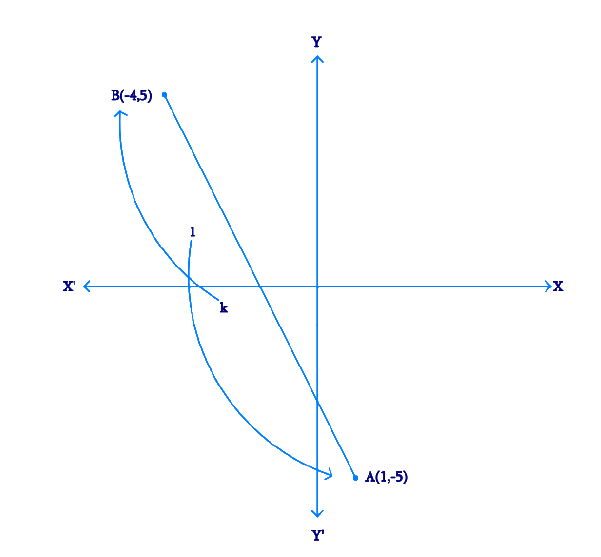# Ex.7.2 Q5 Coordinate Geometry Solution - NCERT Maths Class 10

Go back to  'Ex.7.2'

## Question

Find the ratio in which the line segment joining $$A(1, -5)$$ and $$B(-4, 5)$$ is divided by the $$x$$-axis. Also find the coordinates of the point of division.

Video Solution
Coordinate Geometry
Ex 7.2 | Question 5

## Text Solution

Reasoning:

The coordinates of the point $$P(x, y)$$ which divides the line segment joining the points $$A(x_1, y_1)$$ and $$B(x_2, y_2)$$, internally, in the ratio $$m_1 : m_2$$ is given by the Section Formula.

What is Known?

The $$x$$ and $$y$$ co-ordinates of the line segment which is divided by the $$x$$-axis.

What is Unknown?

The ratio in which the line segment joining $$A(1, -5)$$ and $$B(-4, 5)$$ is divided by the $$x$$-axis and the coordinates of the point of division

Steps:

From the Figure,Given,

• Let the ratio be $$k : 1$$.
• Let the line segment joining $$A(1, -5)$$ and $$B(-4, 5)$$

By Section formula

\begin{align}{{P(x,}}\,{{y)}} & \!=\! \left[\! {\frac{{{{m}}{{{x}}_2} \!+\! {{n}}{{{x}}_1}}}{{{{m}} \!+\! {{n}}}},\frac{{{{m}}{{{y}}_2} \!+\! {{n}}{{{y}}_1}}}{{{{m}} \!+\! {{n}}}}}\! \right] \;\;\dots(1) \\ &\begin{bmatrix}\text{By substituting the values} \\ \text{in Equation (1)}\end{bmatrix} \end{align}

Therefore, the coordinates of the point of division is \begin{align}\left[{\frac{{ - 4k + 1}}{{k + 1}},\;\frac{{5k - 5}}{{k + 1}}} \right]\end{align}

We know that $$y$$-coordinate of any point on $$x$$-axis is $$0$$.

\begin{align}∴\; \frac{{5{{k}} - 5}}{{{{k}} + 1}} &= 0\\\;\;\;5{{k}} - 5 &= 0\\\;\;\;\, \to 5{{k}}& = 5 \\&\!\!\!\!\!\!\!\!\!\!\!\!\!\!\!\!\!\!\! \begin{bmatrix}\text{By Cross Multiplying}\\\text { & Transposing}\end{bmatrix} \\\\{{k}} &= 1\end{align}

Therefore, $$x$$-axis divides it in the ratio $$1:1$$.

Division point

\begin{align}&= \left[ {\frac{{ - 4(1) + 1}}{{1 + 1}},\frac{{5(1) + 5}}{{1 + 1}}} \right]\\ &= \left[ {\frac{{ - 4 + 1}}{2},\frac{{5 + 5}}{2}} \right]\\ &= \left[ {\frac{{ - 3}}{2},0} \right]\end{align}

Learn from the best math teachers and top your exams

• Live one on one classroom and doubt clearing
• Practice worksheets in and after class for conceptual clarity
• Personalized curriculum to keep up with school